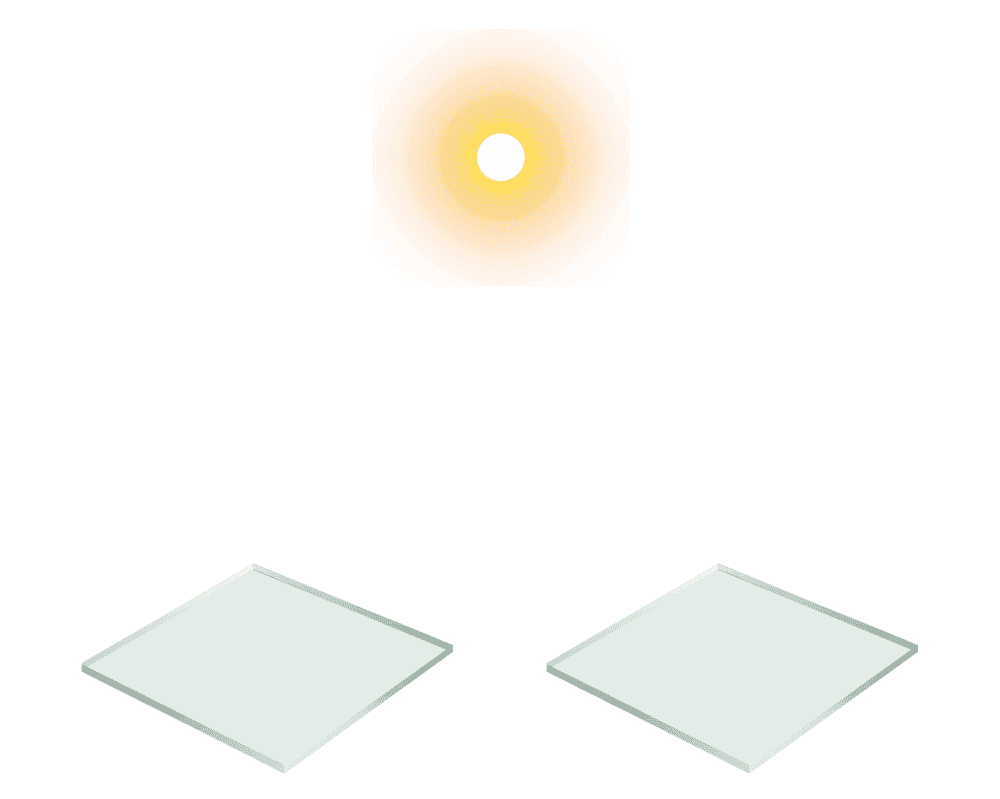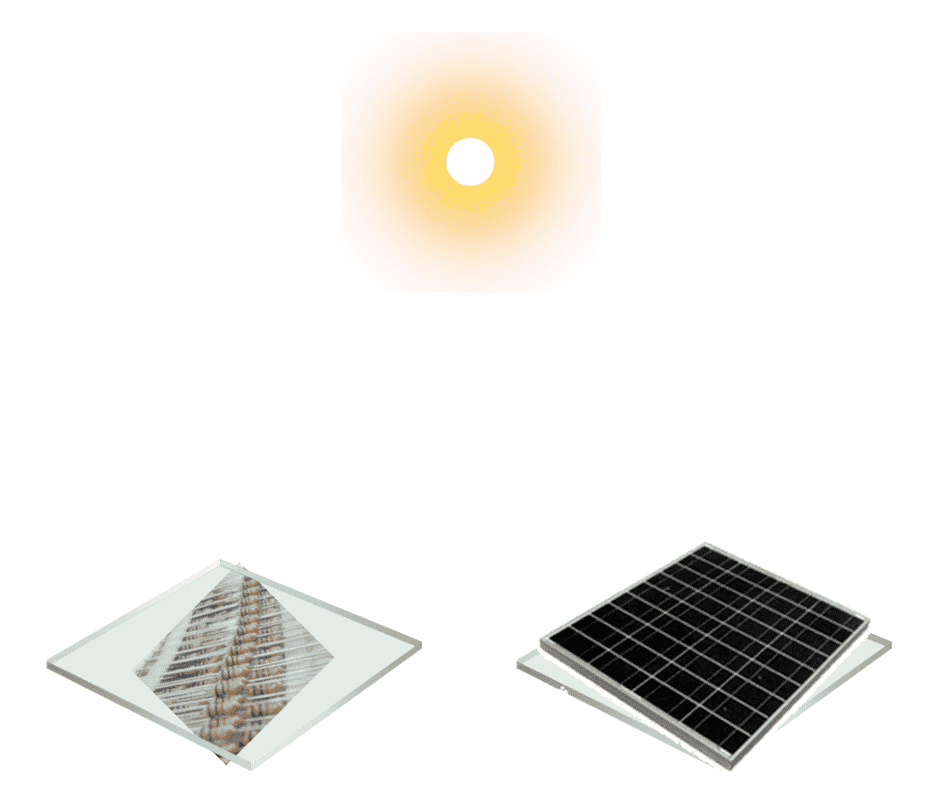# Temperature of a Solar panel in space

nithinyes
Consider two 1 square meter marble slabs each of mass 1 kg floating in space facing the sun such that light from the sun incident perpendicularly on the flat faces.At equilibrium, power received from the sun 'PS' equals the power being lost in the form of radiation 'PR'.

PS = PR (at equilibrium)

For simplicity, assume the equilibrium temperature TE = 300K.

Let us now install a photovoltaic solar panel on the right slab and resistive load on the left slab.

Assume the mass of the photovoltaic panel and resistive load be 1 kg each.

Connect the panel and load using wires and a switch of negligible mass.Let the new equilibrium temperature also be 300 K.

At time t = 0, the pv cell is connected to the load by closing the switch. Let the power being transmitted from pv slab to load slab be 'PPV' and all this power is consumed by the load slab.

So the net power received by load slab is PS + PPV and the net power received by pv slab is PS - PPV.

Since PS + PPV > PS - PPV,

So what happens to the temperature of the slabs?

Last edited by a moderator:

Mentor
So what happens to the temperature of the slabs?
You first: what do you think happens, and why?

•davenn and phinds
nithinyes
You first: what do you think happens, and why?
I think that the power consumed at the load converts into heat and so the temperature of load slab increases.

Coming to pv slab, net power received is reduced due to diversion of some solar power to load power and so the temperature of pv slab decreases.

Am I correct?

Mentor
I think that the power consumed at the load converts into heat and so the temperature of load slab increases.

Coming to pv slab, net power received is reduced due to diversion of some solar power to load power and so the temperature of pv slab decreases.

Am I correct?
Yup!

nithinyes
Yup!
If so, Can we say that photovoltaic cell is a device which reduces its temperature and does useful work on surroundings when it is operating?

DrStupid
If so, Can we say that photovoltaic cell is a device which reduces its temperature and does useful work on surroundings when it is operating?

Yes, but in order to avoid misconception it is better to say that it limits the increase of its temperature by doing work that would otherwise converted into heat.

nithinyes
Yes, but in order to avoid misconception it is better to say that it limits the increase of its temperature by doing work that would otherwise converted into heat.
Can you please explain it a little elaborately in terms of entropy?

DrStupid
Can you please explain it a little elaborately in terms of entropy?

Sunlight (with low entropy) is absorbed by the solar panel and converted into heat (with high entropy). If a part of the absorbed energy is converted into work (without entropy) there is less energy left to heat the panel.

•etotheipi, nithinyes and vanhees71
nithinyes
Sunlight (with low entropy) is absorbed by the solar panel and converted into heat (with high entropy). If a part of the absorbed energy is converted into work (without entropy) there is less energy left to heat the panel.
Thank you

•